# Intermediate Algebra Tutorial 20

Intermediate Algebra
Tutorial 20: Solving Systems of Linear Equations
in Three Variables

WTAMU > Virtual Math Lab > Intermediate AlgebraLearning Objectives

After completing this tutorial, you should be able to:
1. Solve a system of linear equations in three variables by the elimination method.Introduction

In this tutorial we will be specifically looking at systems that have three linear equations and three unknowns.  In Tutorial 19: Solving Systems of Linear Equations in Two Variables we covered systems that have two linear equations and two unknowns.  We will only look at solving them using the elimination method.  Don't get overwhelmed by the length of some of these problems.  Just keep in mind that a lot of the steps are just like the ones from the elimination method of two equations and two unknowns that were covered in Tutorial 19: Solving Systems of Linear Equations in Two Variables, just more of them.Tutorial

System of Linear Equations

A system of linear equations is two or more linear equations that are being solved simultaneously.

In this tutorial, we will be looking at systems that have three linear equations and three unknowns.

Tutorial 19: Solving a System of Linear Equations in Two Variables looked at three ways to solve linear equations in two variables.

Solution of a System

In general, a solution of a system in three variables is an ordered triple (x, y, z) that makes ALL THREE equations true.

In other words, it is what they all three have in common.  So if an ordered triple is a solution to one equation, but not another, then it is NOT a solution to the system.

Note that the linear equations in two variables found in Tutorial 19: Solving a System of Linear Equations in Two Variables graphed to be a line on a two dimensional Cartesian coordinate system.  If you were to graph (which you won't be asked to do here) a linear equation in three variables you would end up with a figure of a plane in a three dimensional coordinate system.  An example of what a plane would look like is a floor or a desk top.

A consistent system is a system that has at least one solution.

An inconsistent system is a system that has no solution.

The equations of a system are dependent if ALL the solutions of one equation are also solutions of the other two equations.  In other words, they end up being the same line.

The equations of a system are independent if they do not share ALL solutions.  They can have one point in common, just not all of them.

There are three possible outcomes that you may encounter when working with these systems:

One Solution
If the system in three variables has one solution, it is an ordered triple (x, y, z) that is a solution to ALL THREE equations.  In other words, when you plug in the values of the ordered triple, it makes ALL THREE equations TRUE.

If you do get one solution for your final answer, is this system consistent or inconsistent?
If you said consistent, give yourself a pat on the back!

If you do get one solution for your final answer, would the equations be dependent or independent?
If you said independent, you are correct!

No Solution
If the three planes are parallel to each other, they will never intersect.  This means they do not have any points in common.  In this situation, you would have no solution.

If you get no solution for your final answer, is this system consistent or inconsistent?
If you said inconsistent, you are right!

If you get no solution for your final answer, would the equations be dependent or independent?
If you said independent, you are correct!

Infinite Solutions
If the three planes end up lying on top of each other, then there is an infinite number of solutions.  In this situation, they would end up being the same plane, so any solution that would work in one equation is going to work in the other.

If you get an infinite number of  solutions for your final answer, is this system consistent or inconsistent?
If you said consistent you are right!

If you get an infinite number of  solutions for your final answer, would the equations be dependent or independent?
If you said dependent you are correct!

Solving Systems of Linear
Equations in Three Variables
Using the Elimination Method

Note that there is more than one way that you can solve this type of system.  Elimination (or addition) method is one of the more common ways of doing it.  So I choose to show it this way.

If you have another way of doing it, by all means, do it your way, then you can check your final answers with mine.  No matter which way you choose to do it, if you are doing it correctly, the answer is going have to be the same.

Step 1: Simplify and put all three equations in the form Ax + By  + Cz = D if needed.

This would involve things like removing ( ) and  removing fractions.

To remove ( ): just use the distributive property.

To remove fractions: since fractions are another way to write division, and the inverse of divide is to multiply, you remove fractions by multiplying both sides by the LCD of all of  your  fractions.

Step 2: Choose to eliminate any one of the variables from any pair of equations.

This works in the same manner as eliminating a variable with two linear equations and two variables as shown in Tutorial 19: Solving a System of Linear Equations in Two Variables.

At this point, you are only working with two of your equations.  In the next step you will incorporate the third equation into the mix.

Looking ahead, you will be adding these two equations together.  In that process you need to make sure that one of the variables drops out, leaving one equation and two unknowns.  The only way you can guarantee that is if you are adding opposites.  The sum of opposites is 0.

It doesn't matter which variable you choose to drop out.  You want to keep it as simple as possible.  If a variable already has opposite coefficients than go right to adding the two equations together.  If they don't, you need to multiply one or both equations by a number that will create opposite coefficients in one of your variables.  You can think of it like a LCD.  Think about what number the original coefficients both go into and multiply each separate equation accordingly.  Make sure that one variable is positive and the other is negative before you add.

For example, if you had a 2x in one equation and a 3x in another equation, you could multiply the first equation by 3 and get 6x and the second equation by -2 to get a -6x.  So when you go to add these two together they will drop out.

Step 3:  Eliminate the SAME variable chosen in step 2 from any other pair of equations, creating a system of two equations and 2 unknowns.

Basically, you are going to do another elimination step, eliminating the same variable we did in step 2, just with a different pair of equations.  For example,  if you used equations 1 and 3 in step 2, then you can use either 1 and 2 OR 2 and 3 in this step.  As long as you are using a different combination of equations you are ok.  This will get that third equation into the mix.  We need to do this to give us two equations to go with our two unknowns that are left after the first elimination.

Follow the same basic logic as shown in step 2 above to do this with the same variable to eliminate but with a new pair of equations.

Step 4:  Solve the remaining system found in step 2 and 3, just as discussed in Tutorial 19: Solving a System of Linear Equations in Two Variables.

After steps 2 and 3, there will be two equations and two unknowns which is exactly what was shown how to solve in Tutorial 19: Solving a System of Linear Equations in Two variables.  You can use any method you want to solve it.  Generally, I find it easiest to use the elimination method, if that is what I used in steps 2 and 3.  It is less confusing that way.

When you solve this system that has two equations and two variables, you will have the values for two of your variables.

Remember that if both variables drop out and you have a FALSE statement, that means your answer is no solution.

If both variables drop out and you have a TRUE statement, that means your answer is infinite solutions, which would be the equation of the line.

Step 5:  Solve for the third variable.

If you come up with a value for the two variables in step 4, that means the three equations have one solution.  Plug the values found in step 4 into any of the equations in the problem that have the missing variable in it and solve for the third variable.

Step 6:  Check.

You can plug in the proposed solution into ALL THREE equations.  If it makes ALL THREE equations true then you have your solution to the system.

If it makes at least one of them false, you need to go back and redo the problem.Example 1:    Solve the system.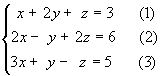Note that the numbers in (  ) are equation numbers.  They will be used throughout the problems for reference purposes.

Basically, we are going to do the same thing we did with the systems of two equations, just more of it.  In other words, we will have to do the elimination twice, to get down to just one variable since we are starting with three variables this time.

Step 1: Simplify and put all three equations in the form Ax + By + Cz = D if needed.

No simplification needed here.  Let's go on to the next step.

Step 2: Choose to eliminate any one of the variables from any pair of equations.

Let’s start by picking our first variable to eliminate.  I’m going to choose y to eliminate.  I need to do this with ANY pair of equations.

Let’s first eliminate y using the first and second equations.  This process is identically to how we approached it with the systems found in Tutorial 19: Solving a System of Linear Equations in Two variables

If I multiply 2 times the second equation, then the y terms will be opposites of each other and ultimately drop out.

Multiplying 2 times the second equation and then adding that to the first equation we get: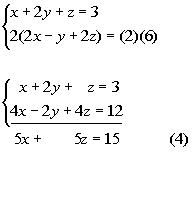*Mult. eq. (2) by 2

*y's have opposite coefficients
*y's dropped out

Now we can’t just stop here for of two reasons.  First, we would be stuck because we have one equation and two unknowns.  Second, when we solve a system it has to be a solution of ALL equations involved and we have not incorporated the third equation yet.  Let’s do that now.

Step 3:  Eliminate the SAME variable chosen in step 2 from any other pair of equations creating a system of two equations and 2 unknowns.

We are still going after eliminating y, this time I want to use the second and the third equations.  I could use the first and third, as long as I have not used both of the same ones used in step 2 above.

I picked the second and third on this example because the y terms are already opposites so we don't have to multiply by anything,  we can go straight to adding them together: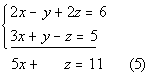*y's have opposite coefficients

*y's dropped out

Step 4:  Solve the remaining system found in step 2 and 3, just as discussed in Tutorial 19: Solving a System of Linear Equations in Two Variables.

Putting the two equations that we have found together, we now have a system of two equations and two unknowns, which we can solve just like the ones shown in Tutorial 19: Solving a System of Linear Equations in Two variables.  You can use either elimination or substitution.  I’m going to go ahead and stick with the elimination method to complete this.

Let’s first put those equations together: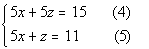*Put equations found in steps 2 and 3
together into one system

Now I’m going to choose x to eliminate.  We can either multiply the first equation by -1 or the second, either way will create opposites in front of the x terms.

I’m going to go ahead and multiply equation (5) by -1 and then add the equations together: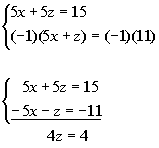*Mult. eq. (5) by -1

*x's have opposite coefficients

*x's dropped out

Solving for z we get: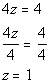*Inverse of mult. by 4 is div. by 4

If we go back one step to the system that had two equations and two variables and put in 1 for z in what is labeled equation 4, we would get: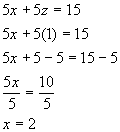*Eq. (4)
*Plug in 1 for z
*Inverse of add 5 is sub. 5

*Inverse of mult. by 5 is div. by 5

Step 5:  Solve for the third variable.

Now we need to go back to the original system and pick any equation to plug in the two known variables and solve for our last variable.

I choose equation (1) to plug in our 2 for x and 1 for z that we found: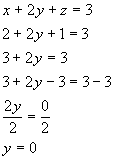*Eq. (1)
*Plug in 2 for x and 1 for z

*Inverse of add 3 is sub. 3

*Inverse of mult. by 2 is div. by 2

Step 6:  Check.

You will find that if you plug the ordered triple (2, 0, 1) into ALL THREE equations of the original system, this is a solution to ALL THREE of them.

(2, 0, 1) is a solution to our system.Example 2:   Solve the system.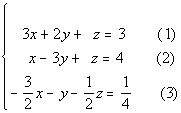Note that the numbers in (  ) are equation numbers.  They will be used throughout the problems for reference purposes.

Step 1: Simplify and put both equations in the form Ax + By + Cz = D if needed.

Unfortunately we have to deal with some fractions in equation (3).
We can take care of them the exact same way they have been dealt with before in equations, by multiplying both sides of equation (3) by it’s LCD, which is 4.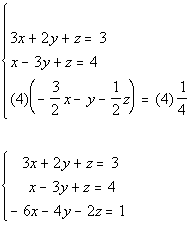*Mult. eq. (3) by LCD 4

Step 2: Choose to eliminate any one of the variables from any pair of equations.

Let’s start by picking our first variable to eliminate.  I’m going to pick z for us to eliminate.  We need to do this with ANY pair of equations.

Let’s first eliminate z using the first and second equations.  This process is identical to how we approached it with the systems found in Tutorial 19: Solving a System of Linear Equations in Two variables.  If  we multiply -1 times the second equation, then the z terms will be opposites of each other and ultimately drop out.

Multiplying -1 times the second equation and then adding that to the first equation we get: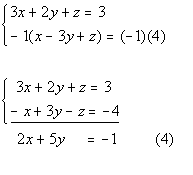*Mult. eq (2) by -1

*z's have opposite coefficients

*z's dropped out

Step 3:  Eliminate the SAME variable chosen in step 2 from any other pair of equations creating a system of two equations and 2 unknowns.

We are still going after eliminating z, this time we want to use the second and the third equations.  We could use the first and third, as long as we have not used both of the same ones used in step 2 above.

It looks like we will have to multiply the first equation by 2, to get opposites on z

Multiplying the first equation by 2 and then adding equations (1) and (3) together we get: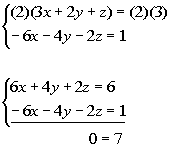*Mult. eq. (2) by 2

*z's have opposite coefficients

*All three variables drop out AND
we have a FALSE statement

Step 4:  Solve the remaining system found in step 2 and 3, just as discussed in Tutorial 19: Solving a System of Linear Equations in Two Variables.

Hey all of our variables disappeared.  What happened???  Just like with two equations and two unknowns shown in Tutorial 19 (Solving a System of Linear Equations in Two variables), when all your variables drop out at any time AND you have a false statement, you end up with no solution for your answer.

Step 5:  Solve for the third variable.

Since we have no solution, there is no value to be found for the third variable.

Step 6:  Check.

No ordered triples to check.Example 3:   Solve the system.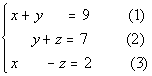Note that the numbers in (  ) are equation numbers.  They will be used throughout the problems for reference purposes.

Step 1: Simplify and put both equations in the form Ax + By + Cz = D if needed.

No simplification needed here.  Let's go on to the next step.

Step 2: Choose to eliminate any one of the variables from any pair of equations.

Note that each equation is missing a variable.  For example equation (1) is missing z.  We can use this to our advantage.  We can use this equation in its original form as an equation with a variable eliminated.

I choose to eliminate z.

Since z is already eliminated from the first equation we will use that first equation in its original form for this step: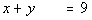*z is already eliminated form eq. (1)

Step 3:  Eliminate the SAME variable chosen in step 2 from any other pair of equations creating a system of two equations and 2 unknowns.

We are still going after eliminating z, this time I want to use the second and the third equations.

Looks like the z’s in the second and third equations already have opposites, so we just need to add them together: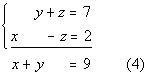*z's have opposite coefficients

*z's dropped out

Step 4:  Solve the remaining system found in step 2 and 3, just as discussed in Tutorial 19: Solving a System of Linear Equations in Two Variables.

Putting the two equations that we have found together we now have a system of two equations and two unknowns.

You can use either elimination or substitution method to solve it.  I’m going to go ahead and stick with the elimination method to complete this.

Let’s first put those equations together: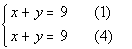*Put equations found in steps 2 and 3
together into one system

Note how we ended up with exactly the same two equations.  It looks like everything is going to drop out again, but this time we will end up with 0 = 0.

Let’s go ahead and show this step by multiplying equation (4) by -1, to get opposite coefficients for x and then add the two equations together.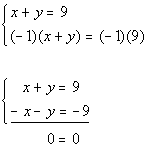*Mult. eq. (4) by -1

*x's have opposite coefficients

*All variables dropped out AND
we have a TRUE statement

As predicted, all variables dropped out AND we have a true statement. This means there is an infinite number of solutions.

Step 5:  Solve for the third variable.

As mentioned above, there is an infinite number of solutions.  So there is no third variable to find.

Step 6:  Check.

There is no ordered triple to check.

{(x, y, z) | x + y = 9}    OR {(x, y, z) | y + z = 7} OR {(x, y, z) | x - z = 2}Practice Problems

These are practice problems to help bring you to the next level.  It will allow you to check and see if you have an understanding of these types of problems. Math works just like anything else, if you want to get good at it, then you need to practice it.  Even the best athletes and musicians had help along the way and lots of practice, practice, practice, to get good at their sport or instrument.  In fact there is no such thing as too much practice.

To get the most out of these, you should work the problem out on your own and then check your answer by clicking on the link for the answer/discussion for that  problem.  At the link you will find the answer as well as any steps that went into finding that answer.Practice Problems 1a - 1b: Solve the system.

1a.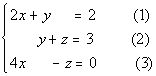1b.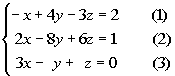Need Extra Help on these Topics?

Go to Get Help Outside the Classroom found in Tutorial 1: How to Succeed in a Math Class for some more suggestions.Last revised on July 10, 2011 by Kim Seward.